# Selina Solutions Concise Maths Class 7 Chapter 15: Triangles Exercise 15B

Selina Solutions Concise Maths Class 7 Chapter 15 Triangles Exercise 15B are designed with the aim of improving problem solving abilities among students. The classification of triangles based on the angles and length of sides are the major concepts discussed under this exercise. Students can cross check their answers and the method of solving using the solutions created by the faculty at BYJU’S. The solved examples create better conceptual knowledge, which are important from the exam perspective. Selina Solutions Concise Maths Class 7 Chapter 15 Triangles Exercise 15B, PDF links are given below for free download.

## Selina Solutions Concise Maths Class 7 Chapter 15: Triangles Exercise 15B Download PDF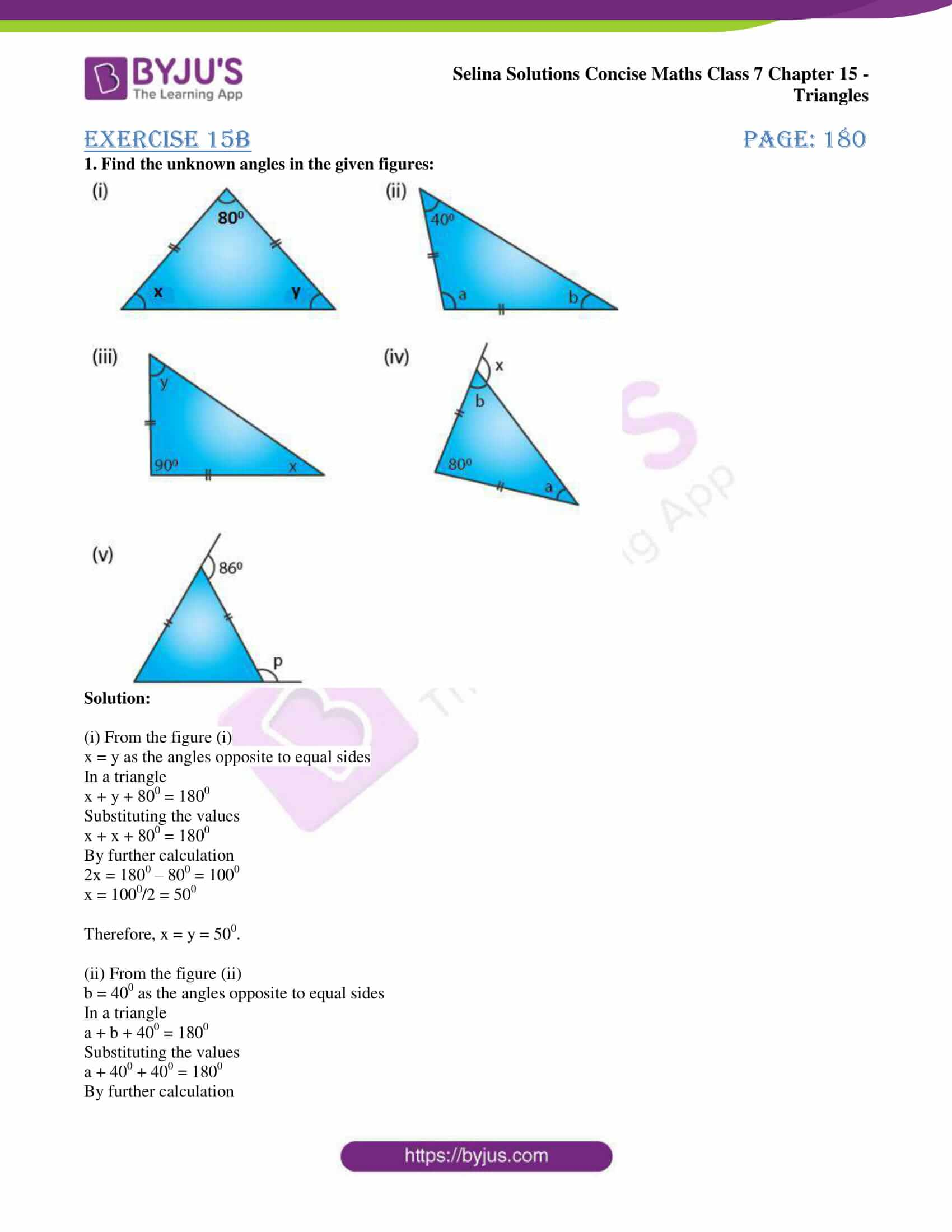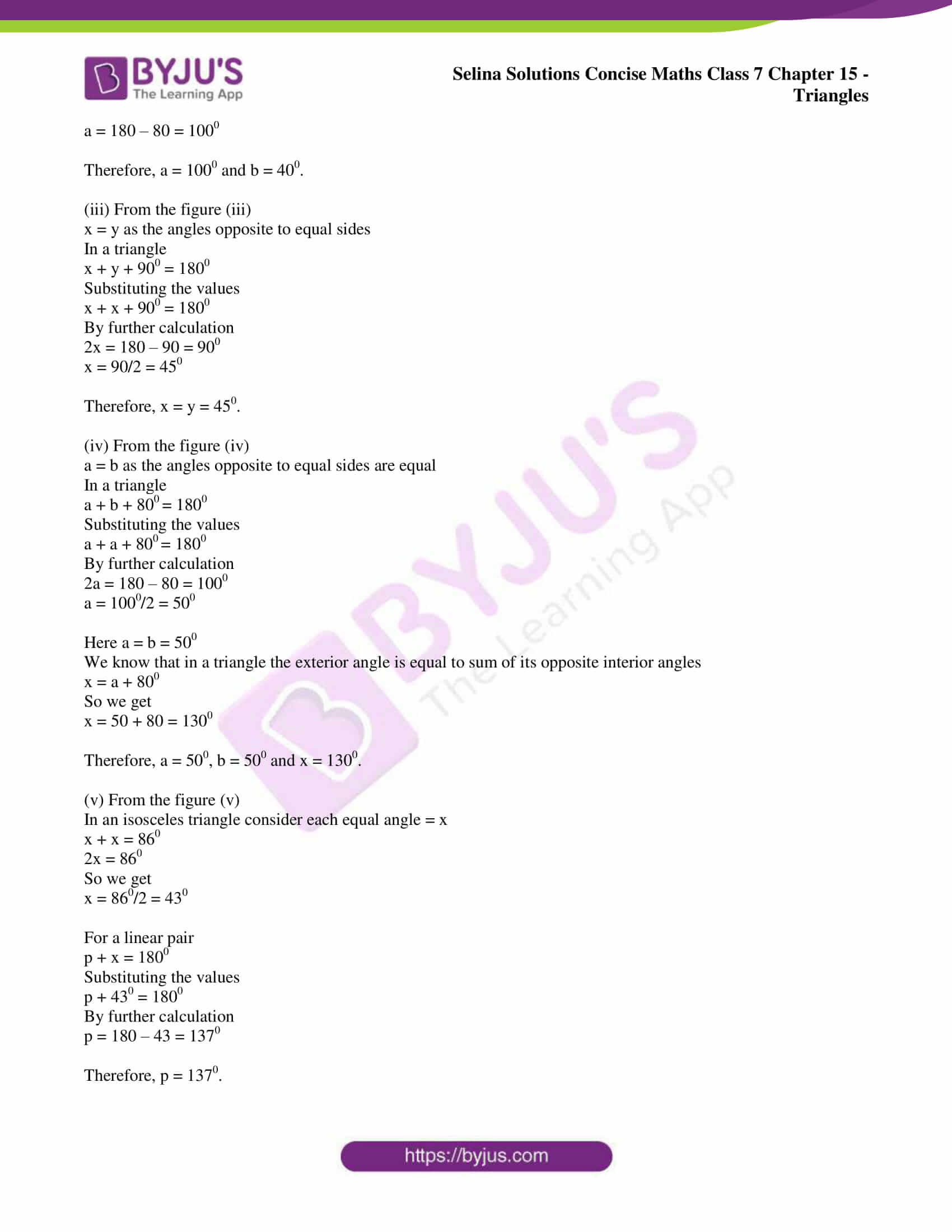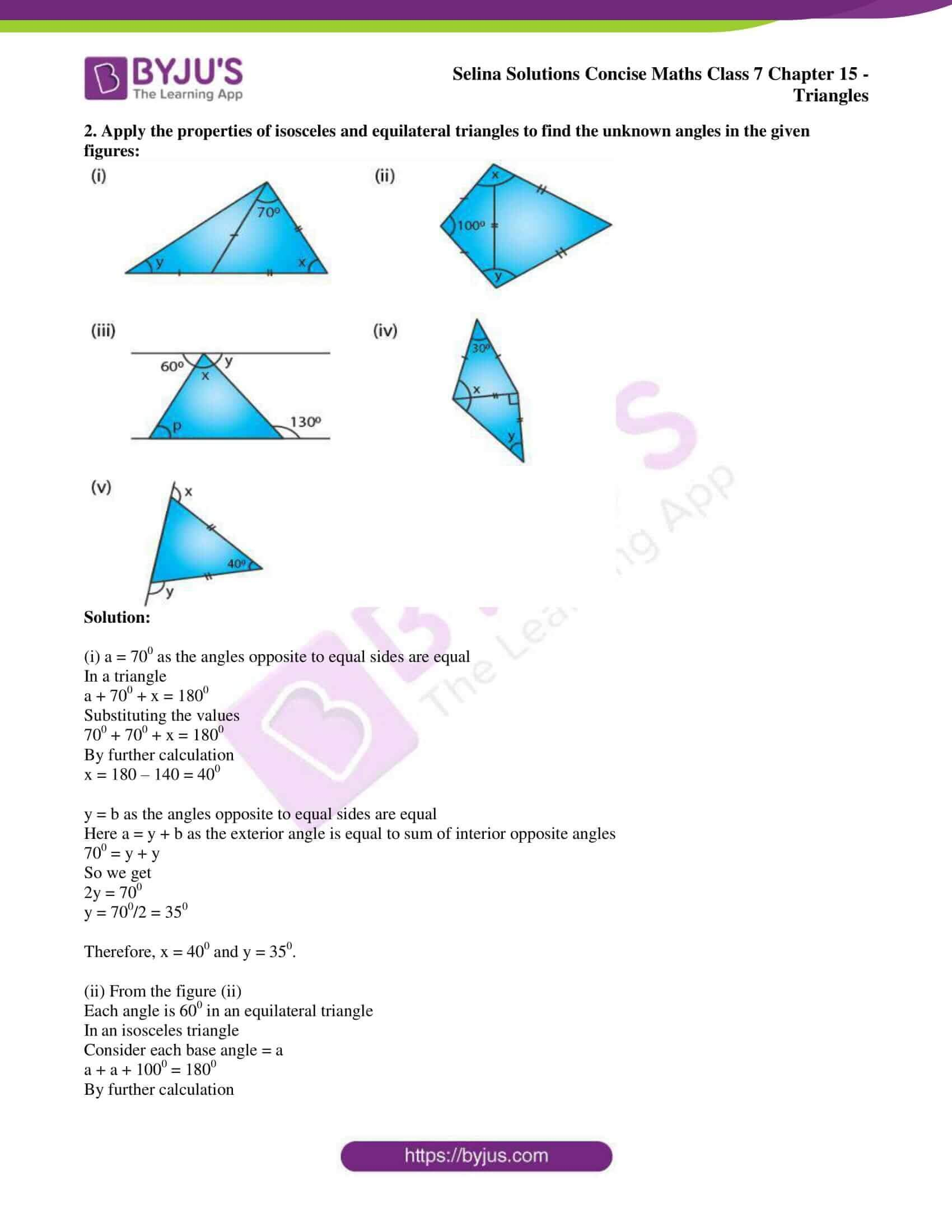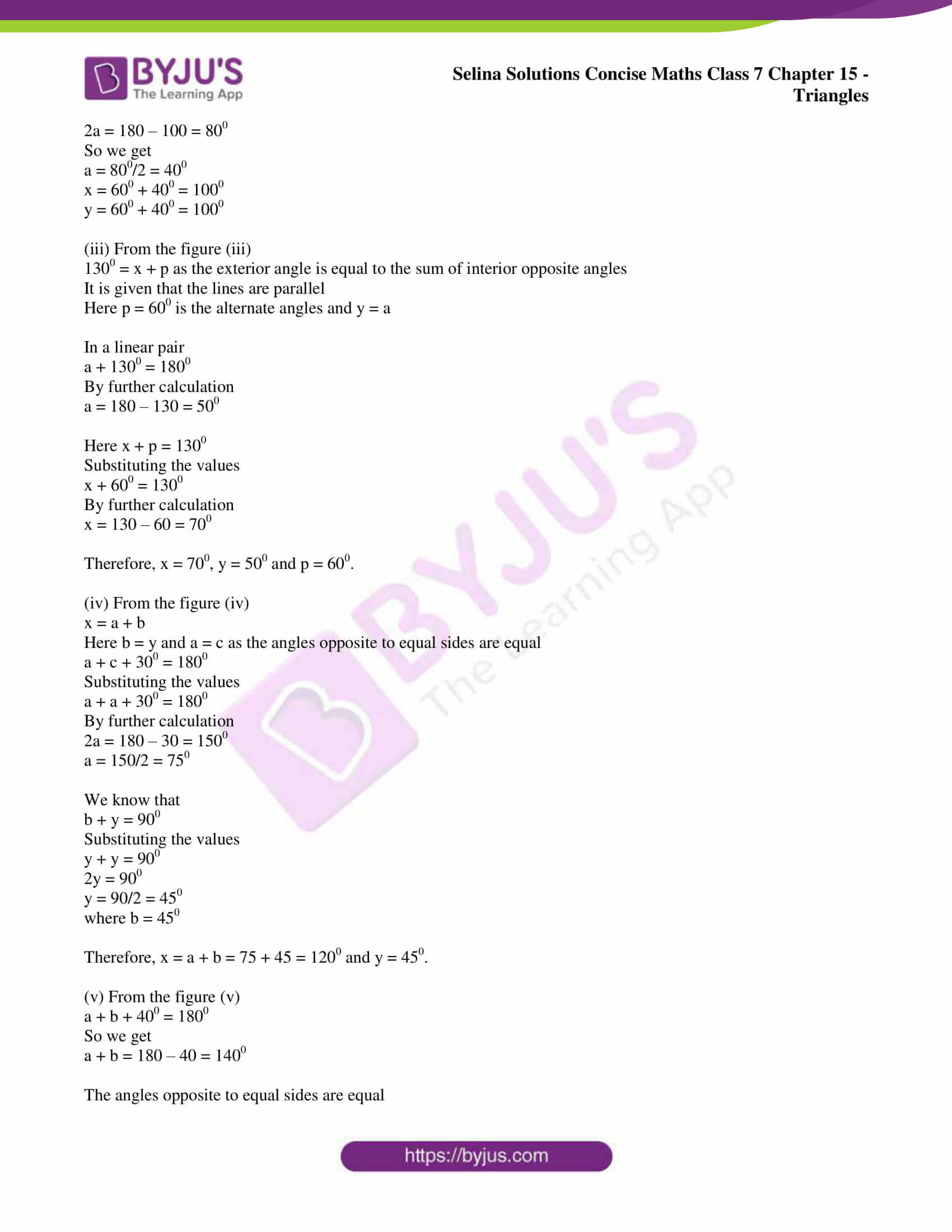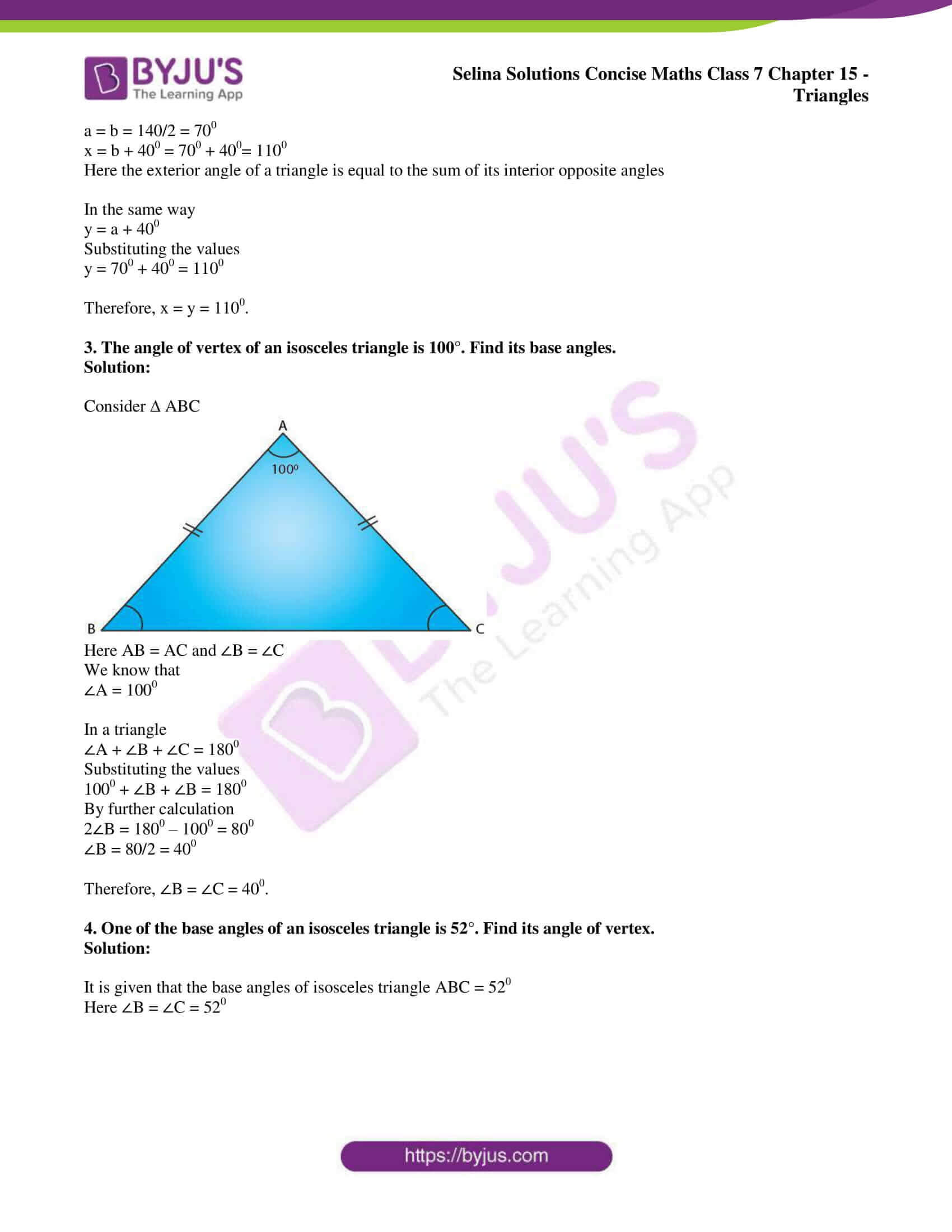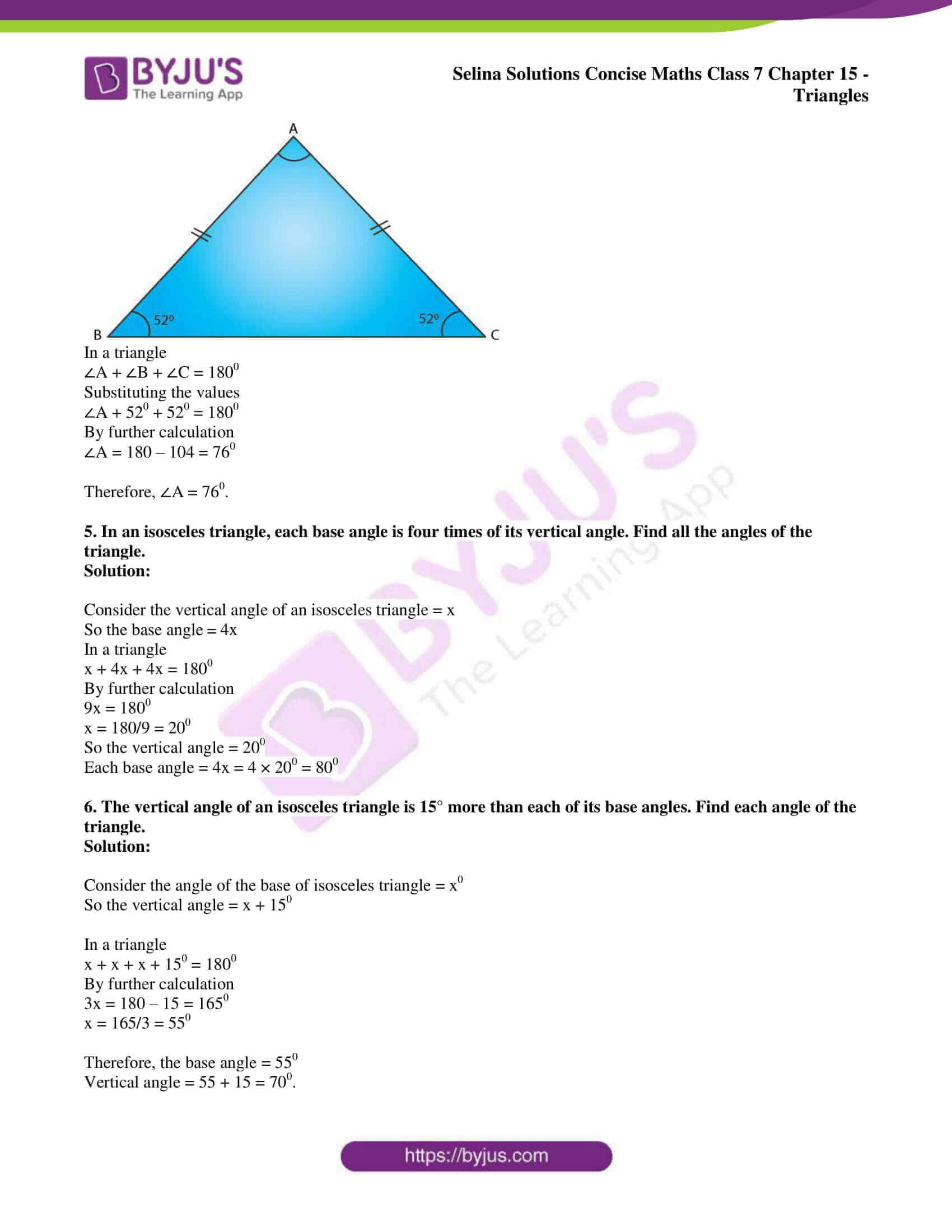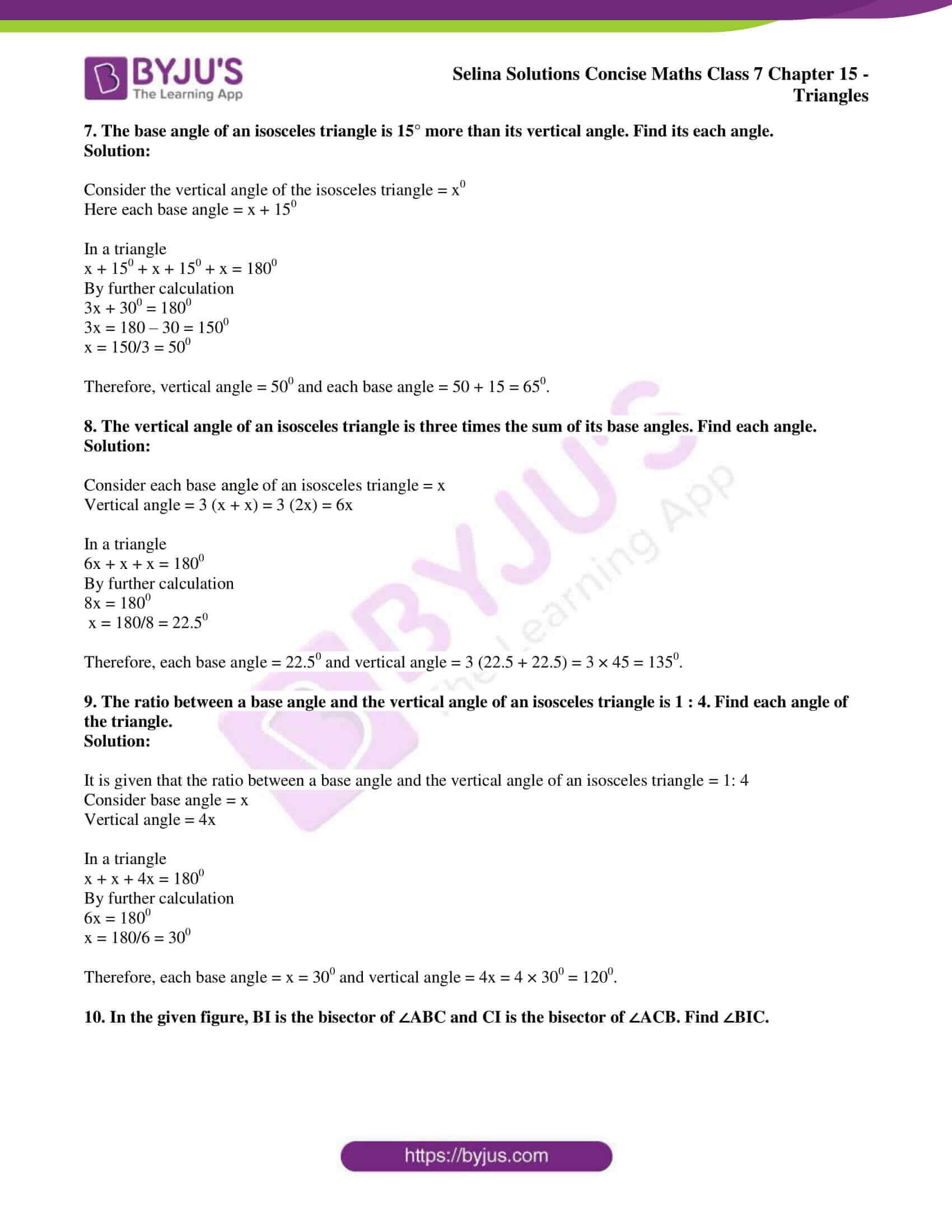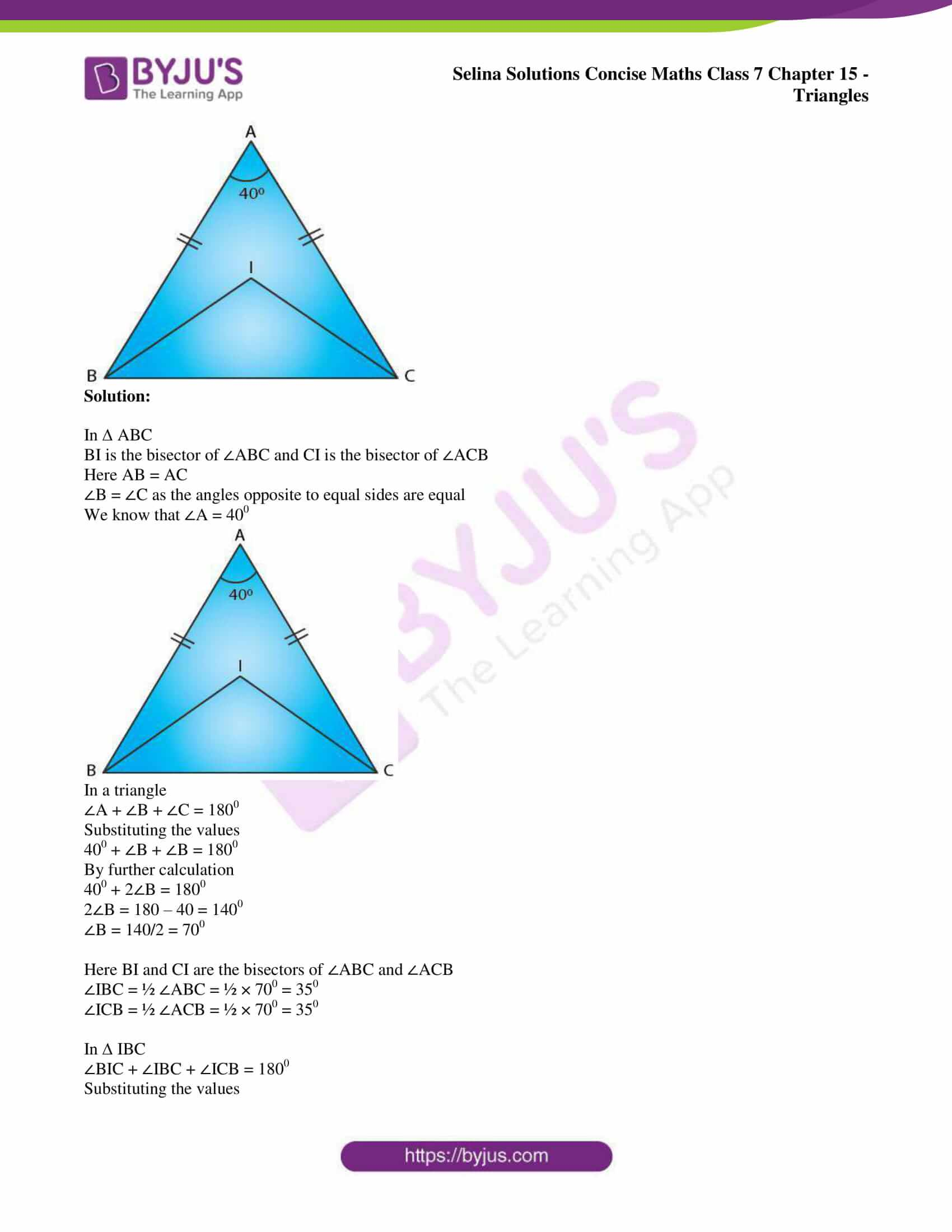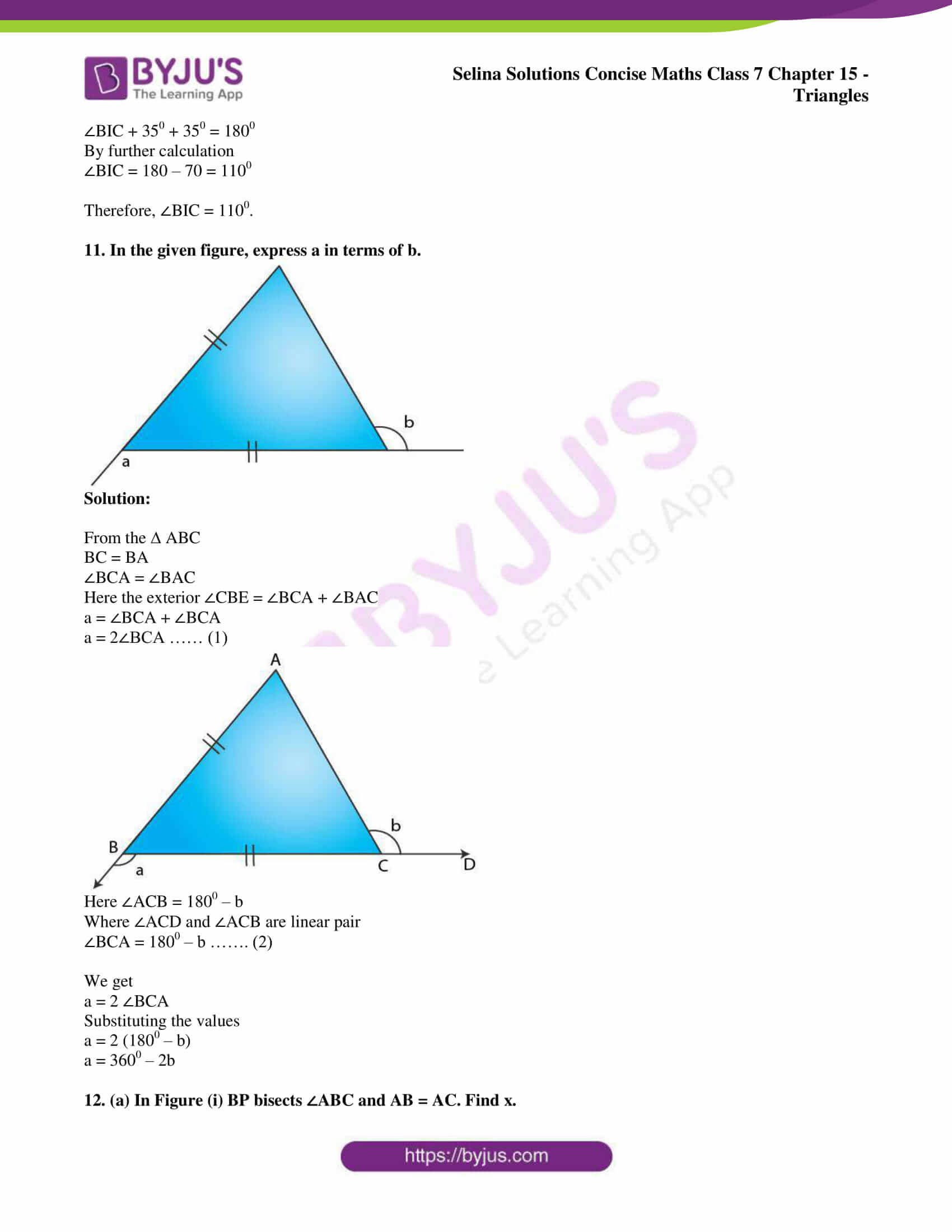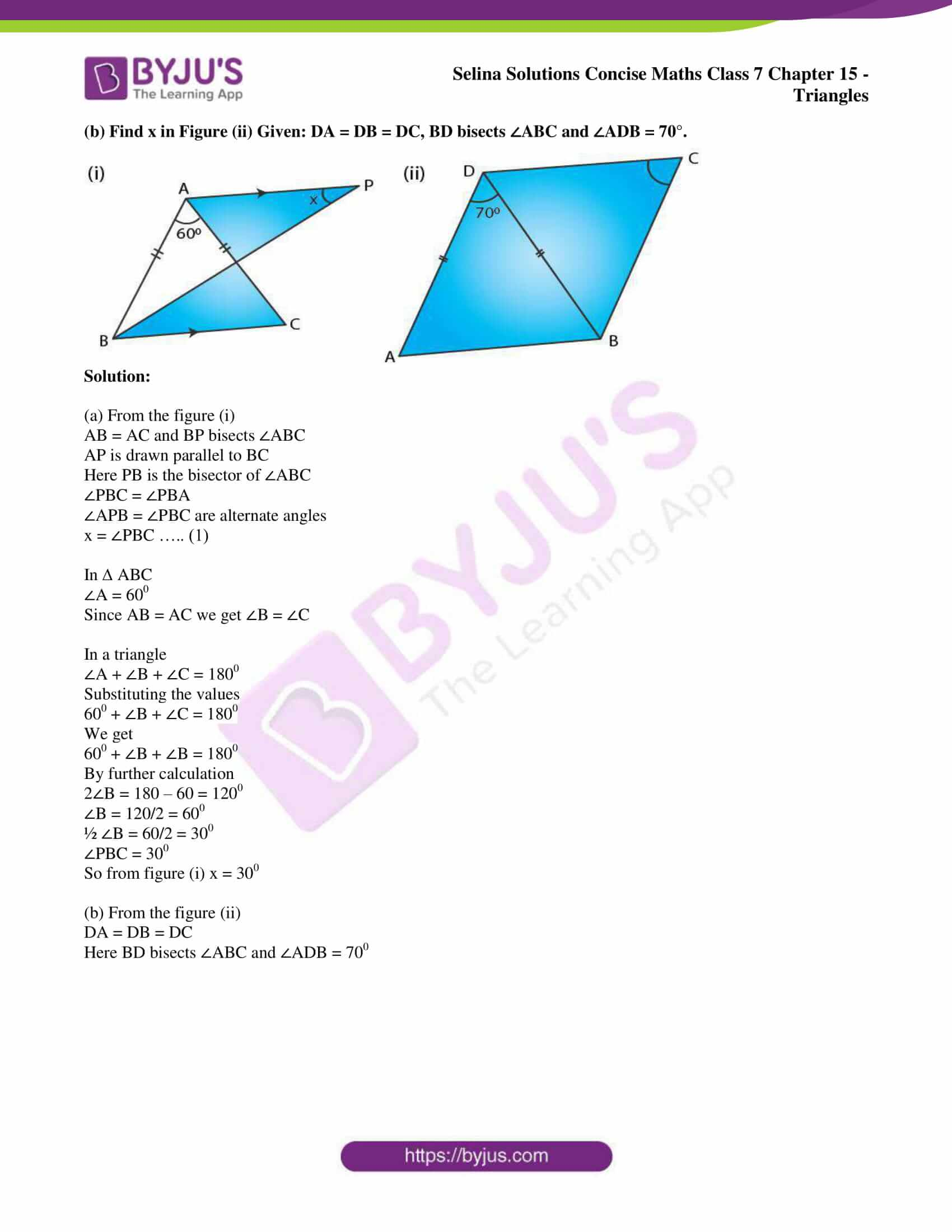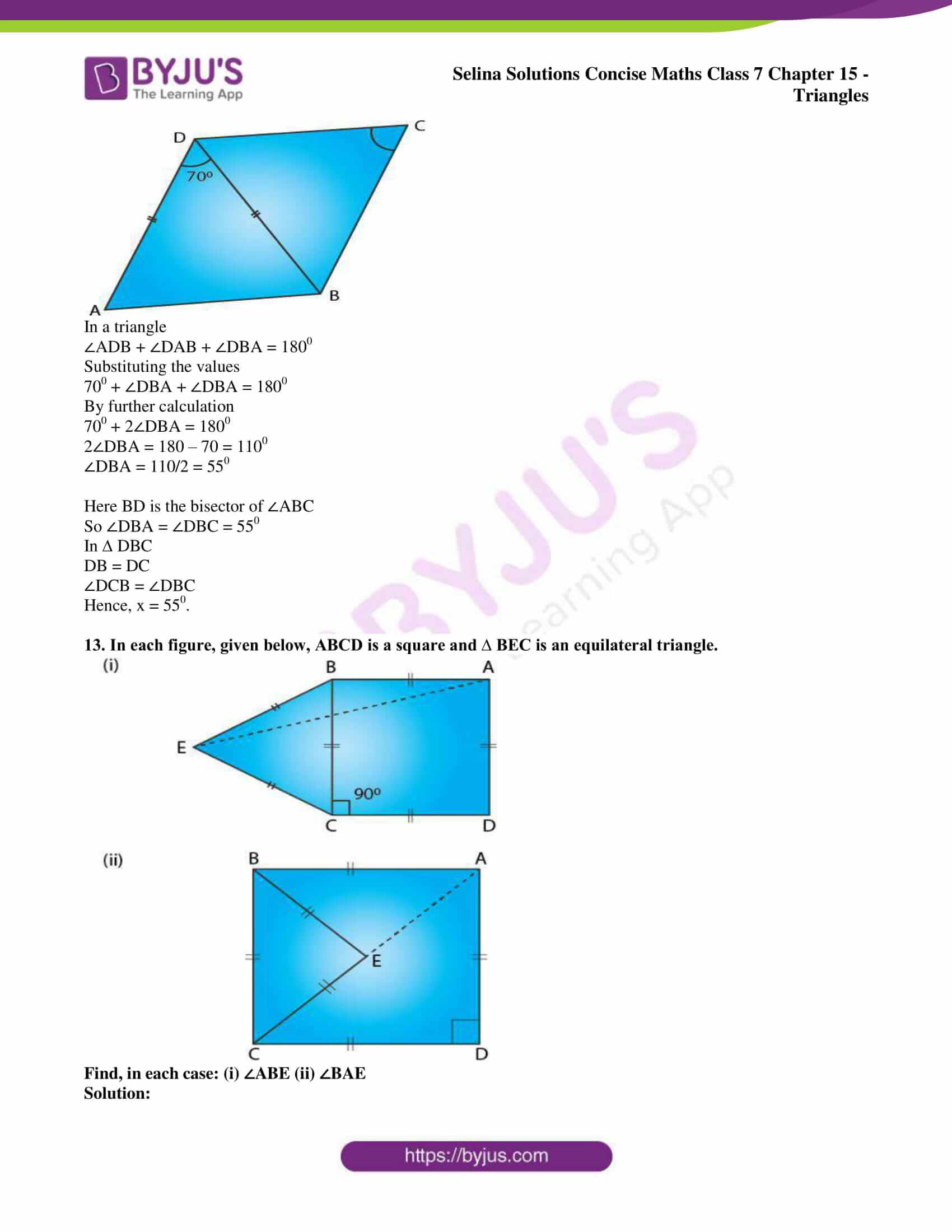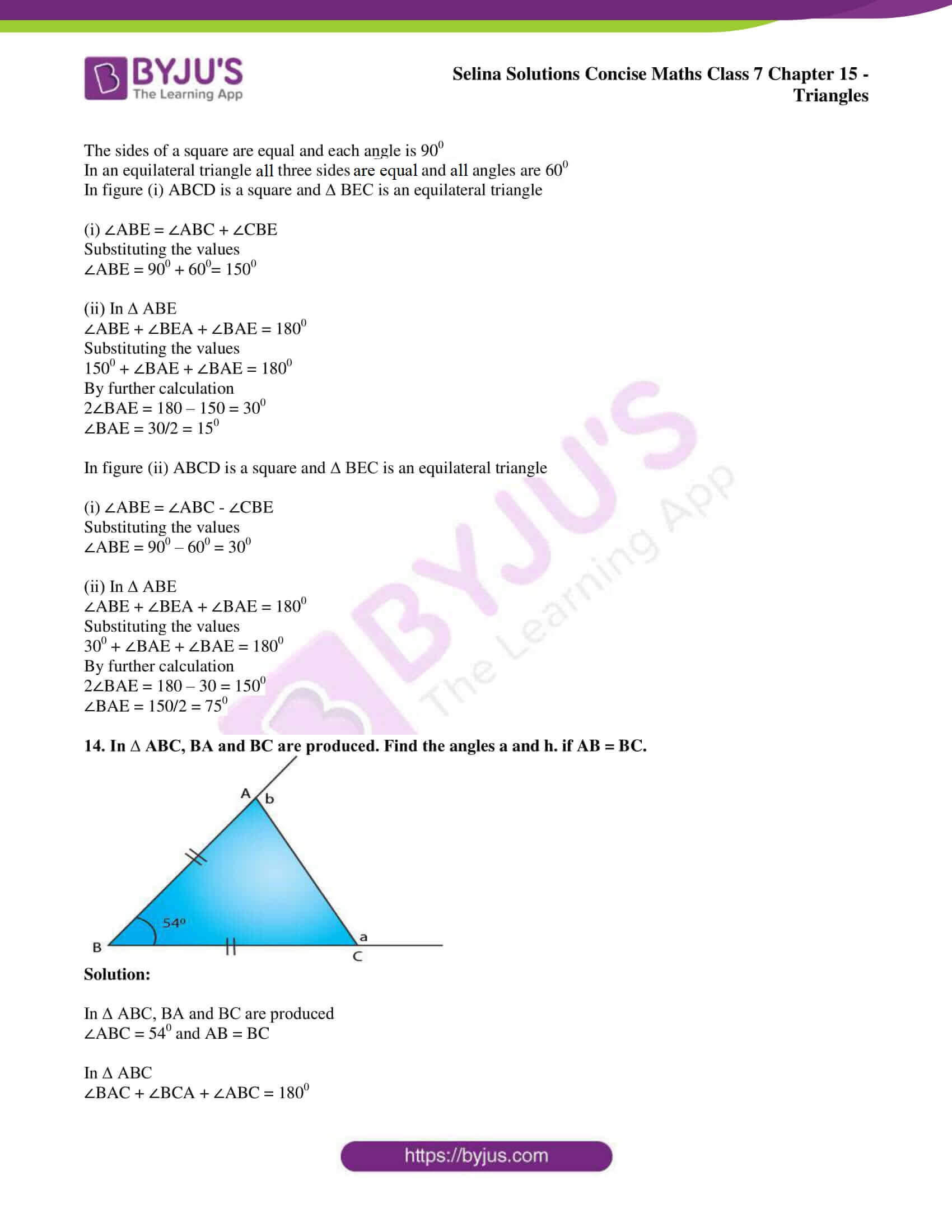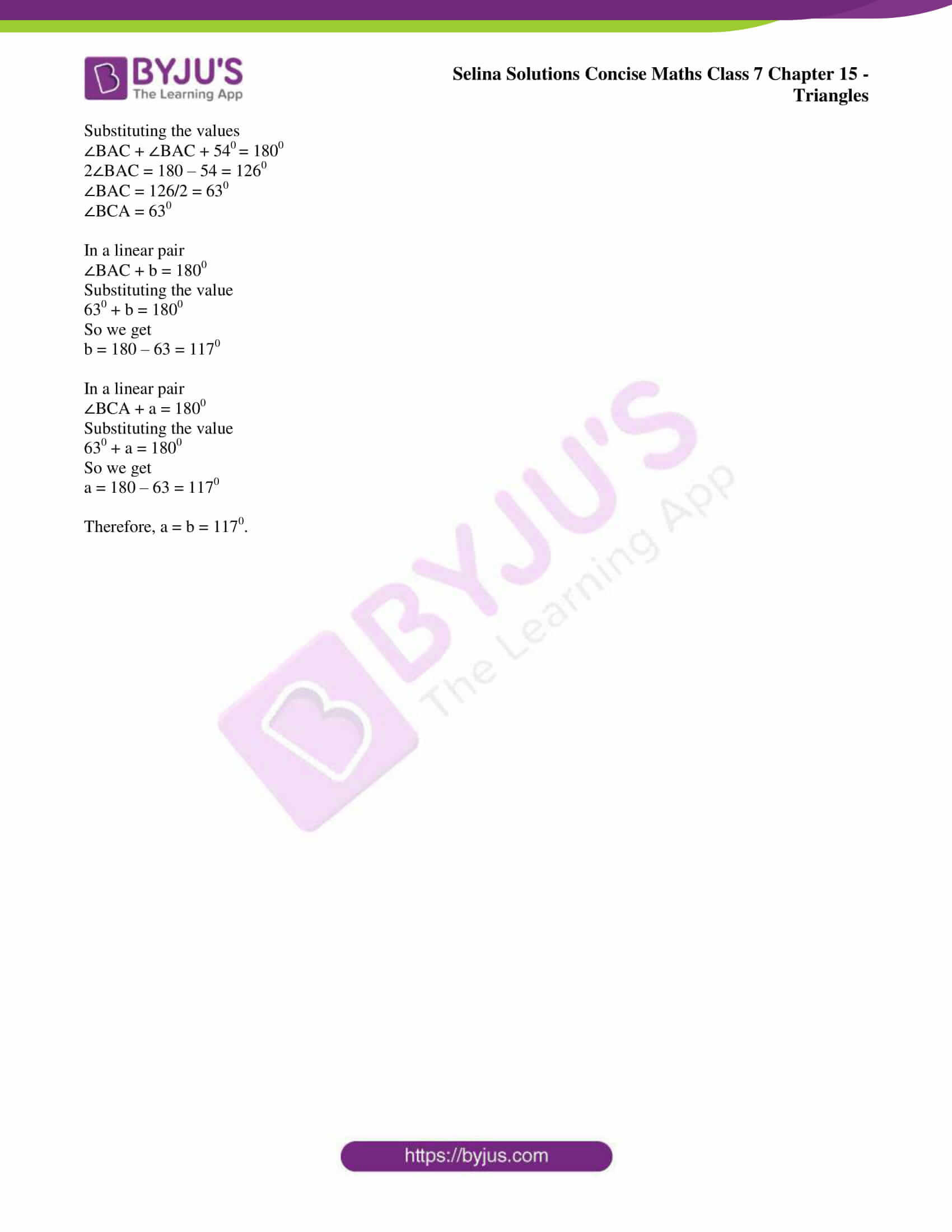### Access other exercises of Selina Solutions Concise Maths Class 7 Chapter 15: Triangles

Exercise 15A Solutions

Exercise 15C Solutions

### Access Selina Solutions Concise Maths Class 7 Chapter 15: Triangles Exercise 15B

#### Exercise 15B page: 180

1. Find the unknown angles in the given figures: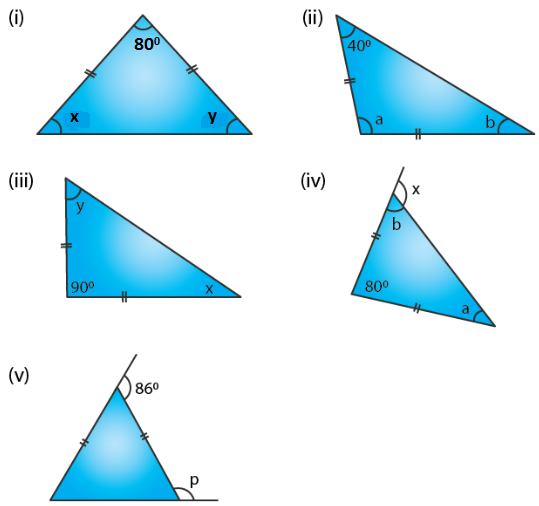Solution:

(i) From the figure (i)

x = y as the angles opposite to equal sides

In a triangle

x + y + 800 = 1800

Substituting the values

x + x + 800 = 1800

By further calculation

2x = 1800 – 800 = 1000

x = 1000/2 = 500

Therefore, x = y = 500.

(ii) From the figure (ii)

b = 400 as the angles opposite to equal sides

In a triangle

a + b + 400 = 1800

Substituting the values

a + 400 + 400 = 1800

By further calculation

a = 180 – 80 = 1000

Therefore, a = 1000 and b = 400.

(iii) From the figure (iii)

x = y as the angles opposite to equal sides

In a triangle

x + y + 900 = 1800

Substituting the values

x + x + 900 = 1800

By further calculation

2x = 180 – 90 = 900

x = 90/2 = 450

Therefore, x = y = 450.

(iv) From the figure (iv)

a = b as the angles opposite to equal sides are equal

In a triangle

a + b + 800 = 1800

Substituting the values

a + a + 800 = 1800

By further calculation

2a = 180 – 80 = 1000

a = 1000/2 = 500

Here a = b = 500

We know that in a triangle the exterior angle is equal to sum of its opposite interior angles

x = a + 800

So we get

x = 50 + 80 = 1300

Therefore, a = 500, b = 500 and x = 1300.

(v) From the figure (v)

In an isosceles triangle consider each equal angle = x

x + x = 860

2x = 860

So we get

x = 860/2 = 430

For a linear pair

p + x = 1800

Substituting the values

p + 430 = 1800

By further calculation

p = 180 – 43 = 1370

Therefore, p = 1370.

2. Apply the properties of isosceles and equilateral triangles to find the unknown angles in the given figures: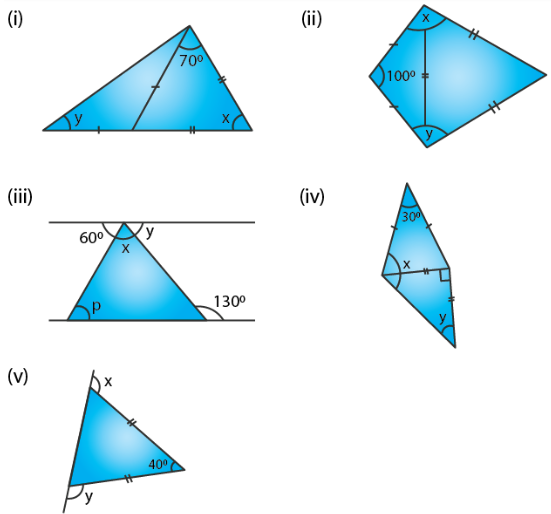Solution:

(i) a = 700 as the angles opposite to equal sides are equal

In a triangle

a + 700 + x = 1800

Substituting the values

700 + 700 + x = 1800

By further calculation

x = 180 – 140 = 400

y = b as the angles opposite to equal sides are equal

Here a = y + b as the exterior angle is equal to sum of interior opposite angles

700 = y + y

So we get

2y = 700

y = 700/2 = 350

Therefore, x = 400 and y = 350.

(ii) From the figure (ii)

Each angle is 600 in an equilateral triangle

In an isosceles triangle

Consider each base angle = a

a + a + 1000 = 1800

By further calculation

2a = 180 – 100 = 800

So we get

a = 800/2 = 400

x = 600 + 400 = 1000

y = 600 + 400 = 1000

(iii) From the figure (iii)

1300 = x + p as the exterior angle is equal to the sum of interior opposite angles

It is given that the lines are parallel

Here p = 600 is the alternate angles and y = a

In a linear pair

a + 1300 = 1800

By further calculation

a = 180 – 130 = 500

Here x + p = 1300

Substituting the values

x + 600 = 1300

By further calculation

x = 130 – 60 = 700

Therefore, x = 700, y = 500 and p = 600.

(iv) From the figure (iv)

x = a + b

Here b = y and a = c as the angles opposite to equal sides are equal

a + c + 300 = 1800

Substituting the values

a + a + 300 = 1800

By further calculation

2a = 180 – 30 = 1500

a = 150/2 = 750

We know that

b + y = 900

Substituting the values

y + y = 900

2y = 900

y = 90/2 = 450

where b = 450

Therefore, x = a + b = 75 + 45 = 1200 and y = 450.

(v) From the figure (v)

a + b + 400 = 1800

So we get

a + b = 180 – 40 = 1400

The angles opposite to equal sides are equal

a = b = 140/2 = 700

x = b + 400 = 700 + 400= 1100

Here the exterior angle of a triangle is equal to the sum of its interior opposite angles

In the same way

y = a + 400

Substituting the values

y = 700 + 400 = 1100

Therefore, x = y = 1100.

3. The angle of vertex of an isosceles triangle is 100°. Find its base angles.

Solution:

Consider ∆ ABC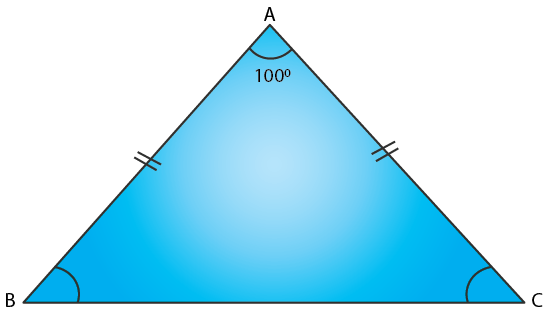Here AB = AC and ∠B = ∠C

We know that

∠A = 1000

In a triangle

∠A + ∠B + ∠C = 1800

Substituting the values

1000 + ∠B + ∠B = 1800

By further calculation

2∠B = 1800 – 1000 = 800

∠B = 80/2 = 400

Therefore, ∠B = ∠C = 400.

4. One of the base angles of an isosceles triangle is 52°. Find its angle of vertex.

Solution:

It is given that the base angles of isosceles triangle ABC = 520

Here ∠B = ∠C = 520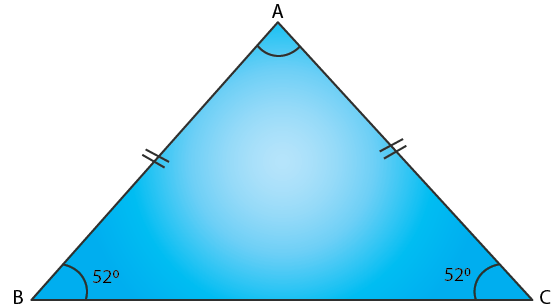In a triangle

∠A + ∠B + ∠C = 1800

Substituting the values

∠A + 520 + 520 = 1800

By further calculation

∠A = 180 – 104 = 760

Therefore, ∠A = 760.

5. In an isosceles triangle, each base angle is four times of its vertical angle. Find all the angles of the triangle.

Solution:

Consider the vertical angle of an isosceles triangle = x

So the base angle = 4x

In a triangle

x + 4x + 4x = 1800

By further calculation

9x = 1800

x = 180/9 = 200

So the vertical angle = 200

Each base angle = 4x = 4 × 200 = 800

6. The vertical angle of an isosceles triangle is 15° more than each of its base angles. Find each angle of the triangle.

Solution:

Consider the angle of the base of isosceles triangle = x0

So the vertical angle = x + 150

In a triangle

x + x + x + 150 = 1800

By further calculation

3x = 180 – 15 = 1650

x = 165/3 = 550

Therefore, the base angle = 550

Vertical angle = 55 + 15 = 700.

7. The base angle of an isosceles triangle is 15° more than its vertical angle. Find its each angle.

Solution:

Consider the vertical angle of the isosceles triangle = x0

Here each base angle = x + 150

In a triangle

x + 150 + x + 150 + x = 1800

By further calculation

3x + 300 = 1800

3x = 180 – 30 = 1500

x = 150/3 = 500

Therefore, vertical angle = 500 and each base angle = 50 + 15 = 650.

8. The vertical angle of an isosceles triangle is three times the sum of its base angles. Find each angle.

Solution:

Consider each base angle of an isosceles triangle = x

Vertical angle = 3 (x + x) = 3 (2x) = 6x

In a triangle

6x + x + x = 1800

By further calculation

8x = 1800

x = 180/8 = 22.50

Therefore, each base angle = 22.50 and vertical angle = 3 (22.5 + 22.5) = 3 × 45 = 1350.

9. The ratio between a base angle and the vertical angle of an isosceles triangle is 1 : 4. Find each angle of the triangle.

Solution:

It is given that the ratio between a base angle and the vertical angle of an isosceles triangle = 1: 4

Consider base angle = x

Vertical angle = 4x

In a triangle

x + x + 4x = 1800

By further calculation

6x = 1800

x = 180/6 = 300

Therefore, each base angle = x = 300 and vertical angle = 4x = 4 × 300 = 1200.

10. In the given figure, BI is the bisector of ∠ABC and CI is the bisector of ∠ACB. Find ∠BIC.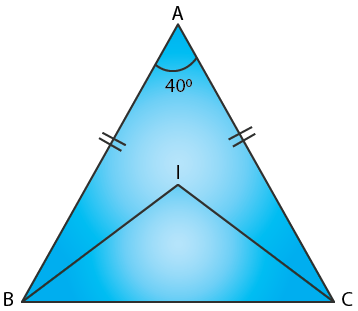Solution:

In ∆ ABC

BI is the bisector of ∠ABC and CI is the bisector of ∠ACB

Here AB = AC

∠B = ∠C as the angles opposite to equal sides are equal

We know that ∠A = 400In a triangle

∠A + ∠B + ∠C = 1800

Substituting the values

400 + ∠B + ∠B = 1800

By further calculation

400 + 2∠B = 1800

2∠B = 180 – 40 = 1400

∠B = 140/2 = 700

Here BI and CI are the bisectors of ∠ABC and ∠ACB

∠IBC = ½ ∠ABC = ½ × 700 = 350

∠ICB = ½ ∠ACB = ½ × 700 = 350

In ∆ IBC

∠BIC + ∠IBC + ∠ICB = 1800

Substituting the values

∠BIC + 350 + 350 = 1800

By further calculation

∠BIC = 180 – 70 = 1100

Therefore, ∠BIC = 1100.

11. In the given figure, express a in terms of b.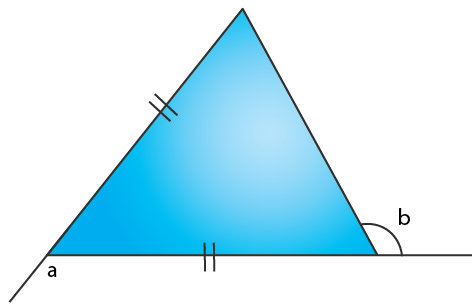Solution:

From the ∆ ABC

BC = BA

∠BCA = ∠BAC

Here the exterior ∠CBE = ∠BCA + ∠BAC

a = ∠BCA + ∠BCA

a = 2∠BCA …… (1)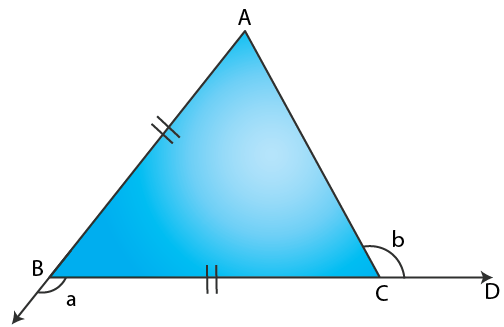Here ∠ACB = 1800 – b

Where ∠ACD and ∠ACB are linear pair

∠BCA = 1800 – b ……. (2)

We get

a = 2 ∠BCA

Substituting the values

a = 2 (1800 – b)

a = 3600 – 2b

12. (a) In Figure (i) BP bisects ∠ABC and AB = AC. Find x.
(b) Find x in Figure (ii) Given: DA = DB = DC, BD bisects ∠ABC and ∠ADB = 70°.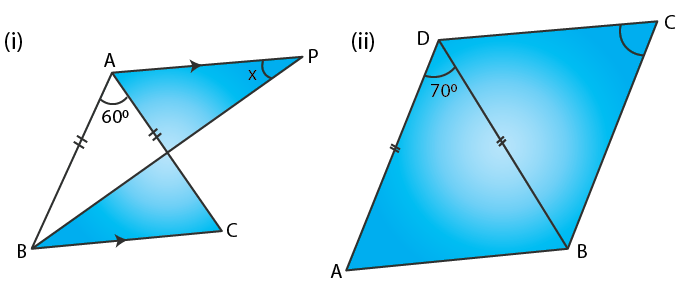Solution:

(a) From the figure (i)

AB = AC and BP bisects ∠ABC

AP is drawn parallel to BC

Here PB is the bisector of ∠ABC

∠PBC = ∠PBA

∠APB = ∠PBC are alternate angles

x = ∠PBC ….. (1)

In ∆ ABC

∠A = 600

Since AB = AC we get ∠B = ∠C

In a triangle

∠A + ∠B + ∠C = 1800

Substituting the values

600 + ∠B + ∠C = 1800

We get

600 + ∠B + ∠B = 1800

By further calculation

2∠B = 180 – 60 = 1200

∠B = 120/2 = 600

½ ∠B = 60/2 = 300

∠PBC = 300

So from figure (i) x = 300

(b) From the figure (ii)

DA = DB = DC

Here BD bisects ∠ABC and ∠ADB = 700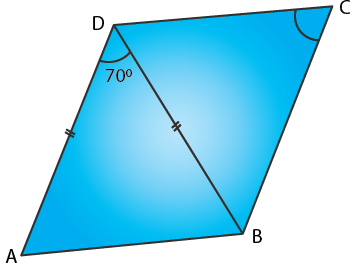In a triangle

∠ADB + ∠DAB + ∠DBA = 1800

Substituting the values

700 + ∠DBA + ∠DBA = 1800

By further calculation

700 + 2∠DBA = 1800

2∠DBA = 180 – 70 = 1100

∠DBA = 110/2 = 550

Here BD is the bisector of ∠ABC

So ∠DBA = ∠DBC = 550

In ∆ DBC

DB = DC

∠DCB = ∠DBC

Hence, x = 550.

13. In each figure, given below, ABCD is a square and ∆ BEC is an equilateral triangle.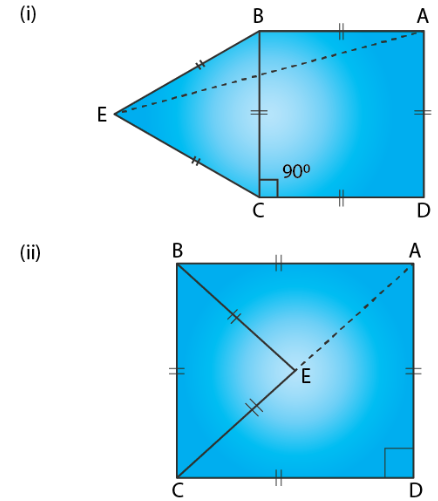Find, in each case: (i) ∠ABE (ii) ∠BAE

Solution:

The sides of a square are equal and each angle is 900

In an equilateral triangle all three sides are equal and all angles are 600

In figure (i) ABCD is a square and ∆ BEC is an equilateral triangle

(i) ∠ABE = ∠ABC + ∠CBE

Substituting the values

∠ABE = 900 + 600= 1500

(ii) In ∆ ABE

∠ABE + ∠BEA + ∠BAE = 1800

Substituting the values

1500 + ∠BAE + ∠BAE = 1800

By further calculation

2∠BAE = 180 – 150 = 300

∠BAE = 30/2 = 150

In figure (ii) ABCD is a square and ∆ BEC is an equilateral triangle

(i) ∠ABE = ∠ABC – ∠CBE

Substituting the values

∠ABE = 900 – 600 = 300

(ii) In ∆ ABE

∠ABE + ∠BEA + ∠BAE = 1800

Substituting the values

300 + ∠BAE + ∠BAE = 1800

By further calculation

2∠BAE = 180 – 30 = 1500

∠BAE = 150/2 = 750

14. In ∆ ABC, BA and BC are produced. Find the angles a and h. if AB = BC.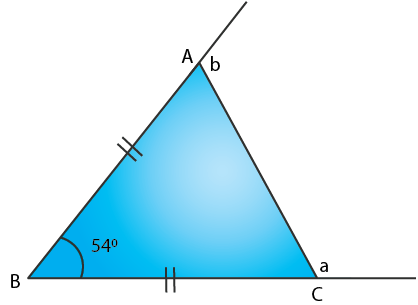Solution:

In ∆ ABC, BA and BC are produced

∠ABC = 540 and AB = BC

In ∆ ABC

∠BAC + ∠BCA + ∠ABC = 1800

Substituting the values

∠BAC + ∠BAC + 540 = 1800

2∠BAC = 180 – 54 = 1260

∠BAC = 126/2 = 630

∠BCA = 630

In a linear pair

∠BAC + b = 1800

Substituting the value

630 + b = 1800

So we get

b = 180 – 63 = 1170

In a linear pair

∠BCA + a = 1800

Substituting the value

630 + a = 1800

So we get

a = 180 – 63 = 1170

Therefore, a = b = 1170.